# Wema Bank Exites Investors with 3k Cash Dividend after Long years of Zero IncentivesCoy: Wema Bank Plc

Current Market Price: N0.77

NPL RATIIO:

Year High: N1.05

Year Low: N 0.55

Fair Value: N2.07

By: Jeariogbe Tunde Segun (Equity Analyst)

Key Financial Ratios

• This report observed the full year financial performance indices of Wema Bank Plc for the year ended, 31st December, 2018; it compare same with the full year financial statistics released in 2017 to establish growth and project future expectations
• Having maintained positive retained earnings stance through and through, the financial institution finally rewards its investors with a cash dividend after a long wait of no incentives.
• The proposed 3k cash dividend is same as 3.90% yield of the price of each units of Wema Bank’s share on the floor of Nigerian Stock Exchange as at the time the result was made available to the investing public.
• Meanwhile, the 9k/share earned through the 2018 financial activities is a yield of 11.20% of the price of each units of Wema Bank’s share on the floor of Nigerian Stock Exchange as at the time the result was made available to the investing public.

Dividend Information

Dividend: 3k

Closure Date: 29th April, 2019

Payment Date: 13th May, 2019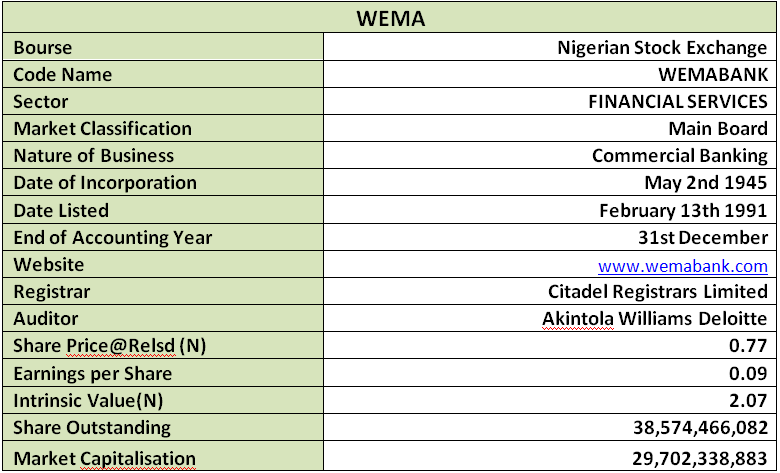Company Figures

• Gross Earnings improved over the comparable year by 9.59% having shifted to N71.529 billion as against the reported N65.268 billion in the corresponding year-2017
• Similarly, Interest Income grew by 8.60% to stand at N57.634 billion compare to N53.073 billion posted in 2017 financial year
• Interest Expenses on the other hand reduced marginally by 8% to stand at N33.306 billion against N30.642 billion in 2017
• Profit before Tax (PBT) builds north by 59.43% as it is currently estimated for N4.797 billion as against the N3.009 billion of similar period in 2017
• Thus, Profit for the period (PAT) followed similar trend, standing at N3.326 billion, this is 47.48% above the N2.255 billion earned through the 2017 financial activities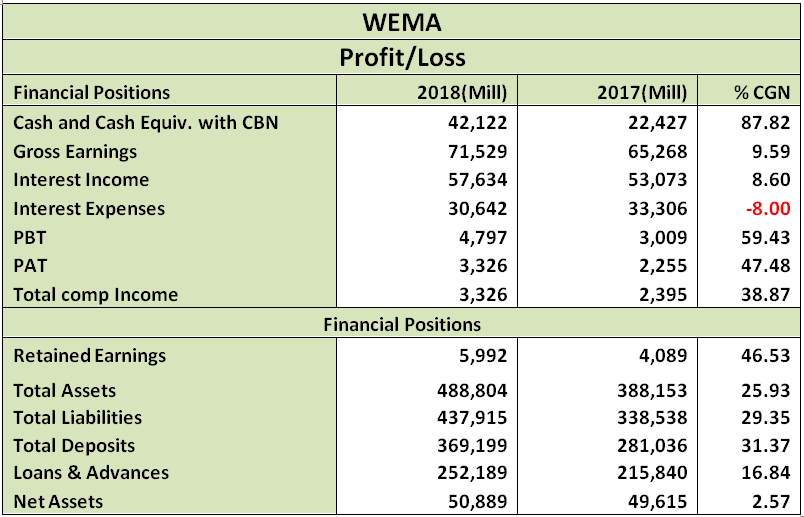• Retained Earnings therefore was built through the year to N5.992 billion, which is 46.53% higher than the N4.089 billion posted for the year ended 31st December, 2017
• Total Assets appreciated to N488.804 billion haven grown by 25.93% above the N388.153 billion valued in 2017 financial year
• Chiefly boosted by growth in the deposits, Total Liabilities stood over that of comparable year by 29.35%; as it is currently estimated at N437.915 billion against N338.538billion
• As noted above, Total Deposit improved by 31.37% to stand at N369.199 billion against N281.036 billion
• Loans & Advances equally improved by 16.84% to stand at N252.189 billion compared to N215.840 billion in 2017
• Meanwhile, due to the fair difference between Total Assets and Total Debt, shareholders’ funds only inched up by 2.57%. Please note that N50.889 billion is the current valuation of this financial index as against N49.615 billion in 2017

Volatility Ratios

• Going by the estimated beta value of Wema Bank Plc, the share is currently enjoying heightened patronage as it currently runs a beta value higher than unity and above industrial average.
• Please understand that our Debt to Equity Ratio is estimated using the entire liability value (which of cause includes the deposit). Thus we have estimated that total liability is same as 8.61 times of the Equity value. This is fairly above the estimated industrial average
• Please note that when we deducted Deposit value from the Total Liabilities, we arrived at Debt to Equity Ratio of 135.03% as against Industrial Average of 187.38%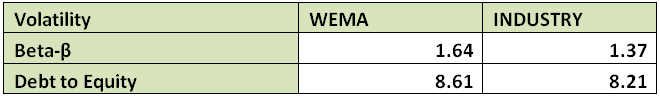Profitability Ratios

• Interest Expense to Gross Earnings dropped by 16.05% from the previously estimated 51.03% to 42.84%
• Profit before Tax Margin (PBT Margin) increased over the previous estimate by 45.48% having moved from 4.61% to 6.71%
• Profit Margin equally grew over the last full year estimate by 34.57%, as it is now estimated at 4.65% as against the previous 3.46%
• Return on Average Equity (ROAE) stood at 43.79% above 2017 estimate. We have currently estimated 6.54% as against 4.55%
• Return on Average Assets equally appreciated by 17.11% to currently stand at the estimate of 0.68% against 0.58% estimated from 2017 financial indices.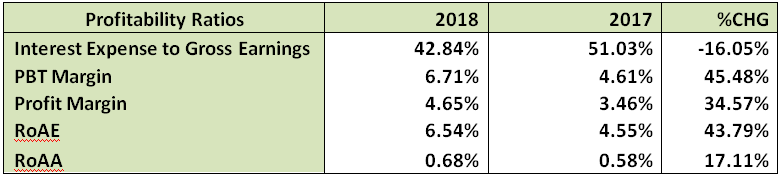Efficiency Ratio

• Gross Earnings to Total Assets is estimated at 14.63% this is 12.97% below the estimate in 2017
• Meanwhile, Gross Earnings to Equity is now 140.56% as against the 131.55% estimated from 2017 full year financial statistics
• It was also established that 68.31% of the Total Deposit was given out as Loan and Advances during the year under estimate; this is; 11.06% lower than the 76.80% given out during through 2017 financial year
• Meanwhile, Loan and Advances is same as 51.59% of the Total Assets, this is 7.22% lower than the 55.61% estimated in 2017.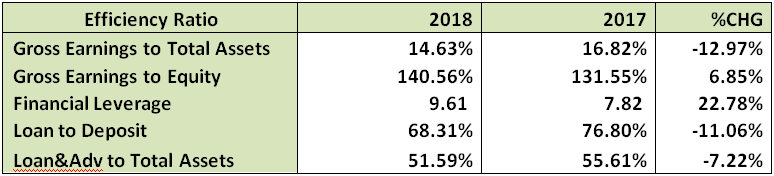Investment Ratios

• The estimated amount earned per units of Wema Bank shares is N0.09, this is 47.48% above the N0.06 earned in 2017 financial year.
• The said earnings per share yielded 11.20% of the current market price on the day the result was made available to the investing public. This is a better yield when compared to the 7.59% achieved last financial year
• PE/Ratio for the period is 8.93x as against 13.17x estimated last year.
• As we speak the Book Value of Wema Bank is N1.32 fairly same as N1.29 last year. Should we choose to value this equity with this indices, we shall conclude an underpriced position of each units of its shares on the floor of the exchange
• Further confirming the underpriced state of Wema Bank Shares is the Price to Book Value ratio that stood below unity.
• Opex Margin was grown by 11.03% having moved to 41.02% from 45.55%
• The N0.03 proposed as dividend for the year is same as 34.79% of the amount earned per unit shares of Wema Bank Plc through the 2018 financial activities. In other words, Retention Ratio is same as 65.21%. In our opinion this is good for a growth stock
• We have also estimated dividend sustainable rate of 4.26%.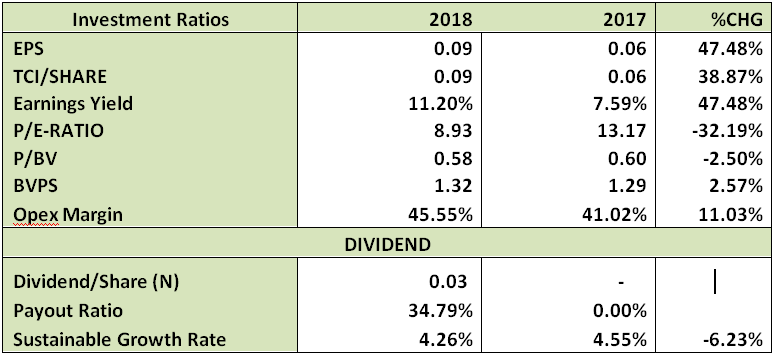Valuation

Through our blend of valuation model, fully considering the non dividend state of the financial institution before this year’s 3k cash dividend, we have valued each units of Wema Bank Plc at N2.07. Thus, considering the current market price of N0.77 (as at the time this report was concluded), we Rate Wema Bank shares a Buy.

# Wema Bank Exites Investors with 3k Cash Dividend after Long years of Zero IncentivesCoy: Wema Bank Plc

Current Market Price: N0.77

NPL RATIIO:

Year High: N1.05

Year Low: N 0.55

Fair Value: N2.07

By: Jeariogbe Tunde Segun (Equity Analyst)

Key Financial Ratios

• This report observed the full year financial performance indices of Wema Bank Plc for the year ended, 31st December, 2018; it compare same with the full year financial statistics released in 2017 to establish growth and project future expectations
• Having maintained positive retained earnings stance through and through, the financial institution finally rewards its investors with a cash dividend after a long wait of no incentives.
• The proposed 3k cash dividend is same as 3.90% yield of the price of each units of Wema Bank’s share on the floor of Nigerian Stock Exchange as at the time the result was made available to the investing public.
• Meanwhile, the 9k/share earned through the 2018 financial activities is a yield of 11.20% of the price of each units of Wema Bank’s share on the floor of Nigerian Stock Exchange as at the time the result was made available to the investing public.

Dividend Information

Dividend: 3k

Closure Date: 29th April, 2019

Payment Date: 13th May, 2019Company Figures

• Gross Earnings improved over the comparable year by 9.59% having shifted to N71.529 billion as against the reported N65.268 billion in the corresponding year-2017
• Similarly, Interest Income grew by 8.60% to stand at N57.634 billion compare to N53.073 billion posted in 2017 financial year
• Interest Expenses on the other hand reduced marginally by 8% to stand at N33.306 billion against N30.642 billion in 2017
• Profit before Tax (PBT) builds north by 59.43% as it is currently estimated for N4.797 billion as against the N3.009 billion of similar period in 2017
• Thus, Profit for the period (PAT) followed similar trend, standing at N3.326 billion, this is 47.48% above the N2.255 billion earned through the 2017 financial activities• Retained Earnings therefore was built through the year to N5.992 billion, which is 46.53% higher than the N4.089 billion posted for the year ended 31st December, 2017
• Total Assets appreciated to N488.804 billion haven grown by 25.93% above the N388.153 billion valued in 2017 financial year
• Chiefly boosted by growth in the deposits, Total Liabilities stood over that of comparable year by 29.35%; as it is currently estimated at N437.915 billion against N338.538billion
• As noted above, Total Deposit improved by 31.37% to stand at N369.199 billion against N281.036 billion
• Loans & Advances equally improved by 16.84% to stand at N252.189 billion compared to N215.840 billion in 2017
• Meanwhile, due to the fair difference between Total Assets and Total Debt, shareholders’ funds only inched up by 2.57%. Please note that N50.889 billion is the current valuation of this financial index as against N49.615 billion in 2017

Volatility Ratios

• Going by the estimated beta value of Wema Bank Plc, the share is currently enjoying heightened patronage as it currently runs a beta value higher than unity and above industrial average.
• Please understand that our Debt to Equity Ratio is estimated using the entire liability value (which of cause includes the deposit). Thus we have estimated that total liability is same as 8.61 times of the Equity value. This is fairly above the estimated industrial average
• Please note that when we deducted Deposit value from the Total Liabilities, we arrived at Debt to Equity Ratio of 135.03% as against Industrial Average of 187.38%Profitability Ratios

• Interest Expense to Gross Earnings dropped by 16.05% from the previously estimated 51.03% to 42.84%
• Profit before Tax Margin (PBT Margin) increased over the previous estimate by 45.48% having moved from 4.61% to 6.71%
• Profit Margin equally grew over the last full year estimate by 34.57%, as it is now estimated at 4.65% as against the previous 3.46%
• Return on Average Equity (ROAE) stood at 43.79% above 2017 estimate. We have currently estimated 6.54% as against 4.55%
• Return on Average Assets equally appreciated by 17.11% to currently stand at the estimate of 0.68% against 0.58% estimated from 2017 financial indices.Efficiency Ratio

• Gross Earnings to Total Assets is estimated at 14.63% this is 12.97% below the estimate in 2017
• Meanwhile, Gross Earnings to Equity is now 140.56% as against the 131.55% estimated from 2017 full year financial statistics
• It was also established that 68.31% of the Total Deposit was given out as Loan and Advances during the year under estimate; this is; 11.06% lower than the 76.80% given out during through 2017 financial year
• Meanwhile, Loan and Advances is same as 51.59% of the Total Assets, this is 7.22% lower than the 55.61% estimated in 2017.Investment Ratios

• The estimated amount earned per units of Wema Bank shares is N0.09, this is 47.48% above the N0.06 earned in 2017 financial year.
• The said earnings per share yielded 11.20% of the current market price on the day the result was made available to the investing public. This is a better yield when compared to the 7.59% achieved last financial year
• PE/Ratio for the period is 8.93x as against 13.17x estimated last year.
• As we speak the Book Value of Wema Bank is N1.32 fairly same as N1.29 last year. Should we choose to value this equity with this indices, we shall conclude an underpriced position of each units of its shares on the floor of the exchange
• Further confirming the underpriced state of Wema Bank Shares is the Price to Book Value ratio that stood below unity.
• Opex Margin was grown by 11.03% having moved to 41.02% from 45.55%
• The N0.03 proposed as dividend for the year is same as 34.79% of the amount earned per unit shares of Wema Bank Plc through the 2018 financial activities. In other words, Retention Ratio is same as 65.21%. In our opinion this is good for a growth stock
• We have also estimated dividend sustainable rate of 4.26%.Valuation

Through our blend of valuation model, fully considering the non dividend state of the financial institution before this year’s 3k cash dividend, we have valued each units of Wema Bank Plc at N2.07. Thus, considering the current market price of N0.77 (as at the time this report was concluded), we Rate Wema Bank shares a Buy.